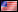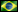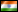## Segmenting LineStrings of arbitrary length with GeoTools

The first and simplest algorithm I will mention deals with segmenting LineStrings so that each segment will have a fixed arbitrary length. Your task is to divide Montreal’s Grand Prix track in segments of 100 meters.

To begin working on this algorithm, you need data, which you can get from the KML (EPSG:4326) of the track you see below:Maven dependencies and repositories

``````
<properties>
<project.build.sourceEncoding>UTF-8</project.build.sourceEncoding>
<geotools.version>12-SNAPSHOT</geotools.version>
</properties>
<dependencies>
<dependency>
<groupId>commons-lang</groupId>
<artifactId>commons-lang</artifactId>
<version>2.6</version>
</dependency>
<dependency>
<groupId>commons-io</groupId>
<artifactId>commons-io</artifactId>
<version>2.4</version>
</dependency>
<dependency>
<groupId>org.geotools</groupId>
<artifactId>gt-xml</artifactId>
<version>\${geotools.version}</version>
</dependency>
<dependency>
<groupId>org.geotools.xsd</groupId>
<artifactId>gt-xsd-kml</artifactId>
<version>\${geotools.version}</version>
</dependency>
<dependency>
<groupId>org.geotools</groupId>
<artifactId>gt-referencing</artifactId>
<version>\${geotools.version}</version>
</dependency>
<dependency>
<groupId>org.geotools</groupId>
<artifactId>gt-render</artifactId>
<version>\${geotools.version}</version>
</dependency>
<dependency>
<groupId>org.geotools</groupId>
<artifactId>gt-epsg-wkt</artifactId>
<version>\${geotools.version}</version>
</dependency>
<dependency>
<groupId>java3d</groupId>
<artifactId>vecmath</artifactId>
<version>1.3.1</version>
</dependency>
</dependencies>

<repositories>
<repository>
<id>maven2-repository.dev.java.net</id>
<name>Java.net repository</name>
</repository>
<repository>
<id>osgeo</id>
<name>Open Source Geospatial Foundation Repository</name>
</repository>
<repository>
<snapshots>
<enabled>true</enabled>
</snapshots>
<id>opengeo</id>
<name>OpenGeo Maven Repository</name>
<url>http://repo.opengeo.org </url>
</repository>
</repositories>
```
```

Importing the example KML into your application

```
```
import java.io.BufferedInputStream;
import java.io.FileInputStream;
import java.io.IOException;
import java.io.InputStream;
import java.net.URISyntaxException;
import java.util.ArrayList;
import java.util.Collection;
import java.util.Collections;
import java.util.Iterator;
import java.util.List;

import javax.xml.parsers.ParserConfigurationException;

import org.geotools.kml.KMLConfiguration;
import org.geotools.referencing.CRS;
import org.geotools.referencing.GeodeticCalculator;
import org.geotools.xml.Parser;
import org.opengis.feature.simple.SimpleFeature;
import org.opengis.referencing.FactoryException;
import org.opengis.referencing.NoSuchAuthorityCodeException;
import org.opengis.referencing.operation.TransformException;
import org.xml.sax.SAXException;

import com.vividsolutions.jts.geom.Coordinate;
import com.vividsolutions.jts.geom.Geometry;
import com.vividsolutions.jts.geom.GeometryFactory;
import com.vividsolutions.jts.geom.LineString;
import com.vividsolutions.jts.geom.Point;
import com.vividsolutions.jts.geom.PrecisionModel;

@SuppressWarnings("unchecked")
private static LineString readGeometryFromKML(String kmlPath) throws IOException, SAXException,
ParserConfigurationException {

Parser parser = new Parser(new KMLConfiguration());
InputStream stream = new BufferedInputStream(new FileInputStream(kmlPath));
LineString track = null;

try {
SimpleFeature feature = (SimpleFeature) parser.parse(stream);
Collection placemarks = (Collection) feature.getAttribute("Feature");
track = (LineString) placemarks.iterator().next().getDefaultGeometry();
} finally {
stream.close();
}

return track;
}
```
```

Now you’re in possession of a LineString object which you will have to divide in segments of 100m. Predictably, there is no method in GeoTools to provide such a specific functionality. What that means is that you’re in for a lot of fun!

The two determinant steps to create this algorithm are:

1. Determining the distance from point A to B
2. Interpolating a point within the line so that you can segment the line at the precise point where the specified distance is reached

Step #1: determining distances in GeoTools

GeoTools has a handy class called GeodeticCalculator for calculating the orthodromic distance between two coordinates in meters. You could also use the orthodromicDistance() method of the JTS class. The most important thing to remember when working with GeodeticCalculator or JTS is the fact that JTS calculates the distance atomically, in just one method. GeodeticCalculator, on the other hand, will require 3 method calls to calculate the same distance. This has implications in multi-threading scenarios depending on how you plan to share the GeodeticCalculator object.

#### Using GeodeticCalculator

```
```
GeodeticCalculator calculator = new GeodeticCalculator(CRS.decode("EPSG:4326")); // KML uses WGS84
Coordinate[] coordinates = track.getCoordinates();

for (int c = 0; c < coordinates.length - 1; c++) {
Coordinate c1 = coordinates[c];
Coordinate c2 = coordinates[c + 1];

calculator.setStartingGeographicPoint(c1.x, c1.y);
calculator.setDestinationGeographicPoint(c2.x, c2.y);

System.out.printf("Segment [%s-%s]. Length: %sm %n", c, c + 1, calculator.getOrthodromicDistance());
}
```
```

#### Using JTS

```
```
CoordinateReferenceSystem crsWGS84 = CRS.decode("EPSG:4326");
Coordinate[] coordinates = track.getCoordinates();

for (int c = 0; c < coordinates.length - 1; c++) {
Coordinate c1 = coordinates[c];
Coordinate c2 = coordinates[c + 1];

System.out.printf("Segment [%s-%s]. Length: %sm %n", c, c + 1, JTS.orthodromicDistance(c1, c2, crsWGS84));
}
```
```

Step #2: interpolating a point within a line

If you can calculate the length of a segment, you can easily sum up the lengths of consecutive segments until you reach a length that is equal or above the specified length — 100m in our case. Your algorithm will eventually reach a segment where its length + the accumulated length is above the required length. You now have to divide your segment at the precise point where you reach 100m. Once again, GeoTools won’t give you this specific functionality out of the box, so you will have to interpolate this point on your own.

One way to know this point is by using the properties of similar triangles.

Let a-b be your line segment. You can easily obtain its 3 important properties:

1. The distance a-b in the X axis (b.x – a.x). Let’s call it “DX”
2. The distance a-b in the Y axis (b.y – a.y). Let’s call it “DY”
3. The distance a-b in meters (Using GeodeticCalculator or JTS). Let’s call it “D”

Once in possession of these 3 properties you can form a triangle and treat your line segment as your hypotenuse.When you divide a line, you can form another triangle in the same fashion, thus creating a child triangle with the same proportions.Now you have everything you need to find the longitude (x) and latitude (y) of the point of segmentation (b):The distance (d) is given by subtracting the required length from the accumulated length. If the required length is 100m and the accumulated length so far is 115m, d = 15m.

Implementation

The code below implements the steps discussed above. Adapt it and optimize it as you see fit!

``````
List<LineString> createSegments(Geometry track, double segmentLength) throws NoSuchAuthorityCodeException,
FactoryException {

GeodeticCalculator calculator = new GeodeticCalculator(CRS.decode("EPSG:4326")); // KML uses WGS84
GeometryFactory geometryFactory = new GeometryFactory(new PrecisionModel(PrecisionModel.FLOATING), 4326);

double accumulatedLength = 0;
List<Coordinate> lastSegment = new ArrayList<Coordinate>();
List<LineString> segments = new ArrayList<LineString>();
Iterator<Coordinate> itCoordinates = coordinates.iterator();

for (int i = 0; itCoordinates.hasNext() && i < coordinates.size() - 1; i++) {
Coordinate c1 = coordinates.get(i);
Coordinate c2 = coordinates.get(i + 1);

calculator.setStartingGeographicPoint(c1.x, c1.y);
calculator.setDestinationGeographicPoint(c2.x, c2.y);

double length = calculator.getOrthodromicDistance();

if (length + accumulatedLength >= segmentLength) {
double offsetLength = segmentLength - accumulatedLength;
double ratio = offsetLength / length;
double dx = c2.x - c1.x;
double dy = c2.y - c1.y;

Coordinate segmentationPoint = new Coordinate(c1.x + (dx * ratio),
c1.y + (dy * ratio));

lastSegment.add(segmentationPoint); // Last point of the segment is the segmentation point

lastSegment = new ArrayList<Coordinate>(); // Resets the variable since a new segment will be built
accumulatedLength = 0D;
} else {
accumulatedLength += length;
}
}

lastSegment.add(coordinates.getLast()); // Because the last one is never added in the loop above

return segments;
}
```
```

Result

Below you can see the end result of segmenting the Montreal Grand Prix track in segments of 100m. Note how the last segment in blue, measuring 26m, is the only segment measuring less than 100m since the total track length is not a multiple of 100. You can download this kml.

This entry was posted by on Monday, May 26th, 2014 at 10:01 pm and is filed under GIS. You can follow any responses to this entry through the RSS 2.0 feed. You can leave a response, or trackback from your own site.

## 5 Responses to “Segmenting LineStrings of arbitrary length with GeoTools”

1.Peter on January 13th, 2015 at 9:49 pm

This is just what I am looking for. Thank you!

I tried cobbling something from what you’ve posted, but it seems there’s a bit more to it, at least with respect to which imports need to be used.

Is there a repository somewhere where I can take a look at the code in context with the needed imports etc?

thank you Dalton!

2.Dalton Filho on January 14th, 2015 at 3:22 pm

Hello Peter,

Sorry for that! GeoTools has dozens of sub-projects. Tracking the library of each and every class is very tricky indeed.

I have now included the Maven dependencies and repositories.

3.Project Plan: A Simple Driving Simulator | My Blog on November 23rd, 2015 at 7:51 pm

4.seema on November 13th, 2017 at 8:43 am

I tried your OL3+Geoserver animation article,but my animation is not working with OL3 .In Geoserver it will work properly,please help me to solve problem.I am giving here my code:

var layout=new ol.layer.Group({
layers:[
new ol.layer.Image({
extent:[-13884991, 2870341, -7455066, 6338219],
source: new ol.source.ImageWMS({
url:’http://localhost:8080/geoserver/topp/wms/animate’,
params: {
‘TILED’: ‘true’,
‘FORMAT’:’image/gif;subtype=animated’,
‘FORMAT_OPTIONS’:’antialias:none;gif_loop_continuosly:true;gif_frames_delay:1000;dpi:180′,
‘TRANSPARENT’:’true’,
‘REQUEST’:’getMap’,
‘SERVICE’: ‘animate’,
‘VERSION’: ‘1.1.1’,
‘APARAM’:’layers’,
5.Dalton Filho on April 21st, 2018 at 7:30 pm